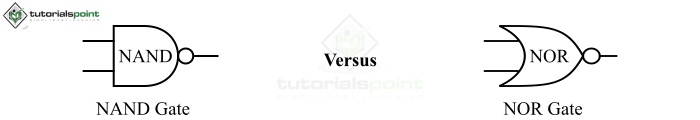# Difference between NAND Gate and NOR Gate

In digital electronics, logic gates are the basic building blocks of all digital circuits that act as the switching devices in the digital circuits. Therefore, a logic gate is a digital circuit used to perform several logical operations in a digital device or system. A logic gate can accept one or multiple inputs but produces only a single output. Where, the output of a logic gate is determined by the combination of input signals. The operation of the logic gates is based on the Boolean algebra.

These days, logic gates are being used in every digital electronic device such as smartphones, laptops, computers, memories, etc. There are many types logic gates available such as AND gate, OR gate, NOT gate, NAND gate, NOR gate, XOR gate, XNOR gate, etc.In this article, we will highlight all the differences between NAND gate and NOR gate. Both the NAND gate and the NOR gate are universal logic gates, which means we can implement any logical expression by using the NAND and NOR gates only. Before getting into the differences, let's start with some basics.

## What is a NAND Gate?

A NAND gate basically a combination of NOT gate and AND gate, i.e. NOT + AND = NAND. Therefore, the NAND gate is a negated version of AND gate.

For a NAND gate, the output of the gate is high (1), when all of its inputs are low (0) or at least one input is low. If it has all the inputs low (0), then the gate's output will be high (1). Hence, from the explanation, it is clear that the NAND gate is an exact inverse of the AND gate.

The logical or Boolean expression of a two input NAND gate is given by,

$$Y\:=\:\overline{\:A\cdot\:B}=\:\:(A\cdot\:B)^\prime$$

Where, Y is the output of the NAND gate and A and B are the binary inputs.

The NAND gate follows the commutative law, i.e.

$$(A\:\cdot\:B)^\prime\:=\:(B\:\cdot\:A)^\prime$$

Hence, from the Boolean expression of the NAND gate, we can see that the output of the NAND gate is obtained by multiplying all the inputs and then by taking the compliment of the multiplied result.

The following is the truth table of a two input NAND gate −

Inputs AND Output
A B A.B Y = (A.B)'
0 0 0 1
0 1 0 1
1 0 0 1
1 1 1 0

NAND gates are used in realizing other logic gates, making flip-flops, registers, burglar alarm circuit, freezer warning buzzer, etc.

## What is a NOR Gate?

The NOR gate is a combination of NOT and OR gates, i.e. OR + NOT = NOR. A NOR gate consists of an OR gate followed by a NOT gate.

For the NOR gate, the output of the gate is high (1), when all its inputs are low (0). In all other cases, it produces a low output. Thus, the NOR gate is nothing but a negated version of the OR gate.

The Boolean expression of a two input NOR gate is given by,

$$Y\:=\:\overline{\:A\:+\:B}=\:\:(A\:+\:B)^\prime$$

Where, Y is the gate's output and A & B are the inputs. Hence, from the Boolean expression of the NOR gate, it is clear that the gate's output can be obtained by the logical addition of all the inputs and then taking the complement of the result of addition.

The following is the truth table of a two input NOR gate −

Inputs OR Output
A B A+B Y = (A+B)'
0 0 0 1
0 1 1 0
1 0 1 0
1 1 1 0

The NOR gate is used in the realization of several combinational and sequential digital circuits like multiplexers, multipliers, counters, etc.

## Difference between NAND Gate and NOR Gate

NAND and NOR gates are types of universal logic gates, however, there are several differences between these that are listed in the following table-

Basis of Difference NAND Gate NOR Gate
Definition A NAND gate is a universal logic gate which performs the negated logical multiplication. A NOR gate is a universal logic gate which performs the negated logical addition.
Implementation NAND gate can be implemented by using an AND gate followed by a NOT gate. NOR gate can be implemented by using an OR gate followed by a NOT gate.
Representation The operation of NAND gate can be represented by the complimented AND operation, i.e. (.)'. The operation of a NOR gate can be represented by the complimented OR operation, i.e. (+)'.
Boolean expression The Boolean expression of a two input NAND gate is given by,

$$Y\:=\:\overline{\:A\:\cdot\:B}=\:\:(A\:\cdot\:B)^\prime$$

The Boolean expression of a two input NOR gate is given by,

$$Y\:=\:\overline{\:A\:+\:B}=\:\:(A\:+\:B)^\prime$$

Low output The NAND gate produces a low (0) output, when all its inputs are high. The NOR gate produces a low (0) output, when all its inputs or at least one input is high (1).
High output The NAND gate produces a high (1) output, when all its inputs or at least one input is low (0). The NOR gate produces a high (1) output, when all its inputs are low (0).
Applications The NAND gate is used in constructing other logic gates, making flip-flops, registers, implementing burglar alarm circuit, freezer warning buzzer, etc. The NOR gate is used in the implementation of various combinational and sequential digital circuits like multiplexers, multipliers, counters, etc.

## Conclusion

The most significant difference between these two gates is that the NAND gate executes a negated logical multiplication, while the NOR gate executes a negated logical addition.

Updated on: 22-Sep-2022

7K+ Views